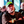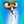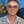0%
0%

# Computer build from first principals

Complete computer build from the logic to the compiler and operating system.

Similar projects worth following
1.2k views
I'm currently working on building a complete computer from the logic up. I will be implementing it 3 ways with bread boards, in Logisim and on an emulator.

Stage 1) Boolean Logic & Arithmetic

Stage 2) Sequential Logic

Stage 3) Machine Language and Computer Architecture

Stage 4) Assembler

Stage 5) Stack Arithmetic, Program Control and high level language design.

Stage 6) Compiler syntax analysis and code generation.

Stage 7) Operating system.

### MUX.jpg

Multiplexer 8 way 16bit

JPEG Image - 29.96 kB - 06/25/2019 at 08:46

### DMUX.jpg

Demultiplexer 8 way

JPEG Image - 13.30 kB - 06/25/2019 at 08:46

### XOR.png

XOR. Nand implementation.

Portable Network Graphics (PNG) - 7.94 kB - 06/25/2019 at 08:47

### SINGLEMUX.jpg

Multiplexer Nand implementation.

JPEG Image - 21.94 kB - 06/25/2019 at 08:47

### SINGLEDMUX.jpg

Demultiplexer Nand implementation.

JPEG Image - 21.69 kB - 06/25/2019 at 08:48

• 1 × ALU 16 Function Arithmetic Logic Unit.

• ### ALU Implemented

Michael Walker06/26/2019 at 06:10 0 comments

```/**
* The ALU (Arithmetic Logic Unit).
* Computes one of the following functions:
* x+y, x-y, y-x, 0, 1, -1, x, y, -x, -y, !x, !y,
* x+1, y+1, x-1, y-1, x&y, x|y on two 16-bit inputs,
* according to 6 input bits denoted zx,nx,zy,ny,f,no.
* In addition, the ALU computes two 1-bit outputs:
* if the ALU output == 0, zr is set to 1; otherwise zr is set to 0;
* if the ALU output < 0, ng is set to 1; otherwise ng is set to 0.
*/

// Implementation: the ALU logic manipulates the x and y inputs
// and operates on the resulting values, as follows:
// if (zx == 1) set x = 0        // 16-bit constant
// if (nx == 1) set x = !x       // bitwise not
// if (zy == 1) set y = 0        // 16-bit constant
// if (ny == 1) set y = !y       // bitwise not
// if (f == 1)  set out = x + y  // integer 2's complement addition
// if (f == 0)  set out = x & y  // bitwise and
// if (no == 1) set out = !out   // bitwise not
// if (out == 0) set zr = 1
// if (out < 0) set ng = 1

CHIP ALU {
IN
x, y,  // 16-bit inputs
zx, // zero the x input?
nx, // negate the x input?
zy, // zero the y input?
ny, // negate the y input?
f,  // compute out = x + y (if 1) or x & y (if 0)
no; // negate the out output?

OUT
out, // 16-bit output
zr, // 1 if (out == 0), 0 otherwise
ng; // 1 if (out < 0),  0 otherwise

PARTS:
// zx
And16(a=x, b=false, out=zxTrue);
Mux16(a=x, b=zxTrue, sel=zx, out=zerox);

// nx
Not16(in=zerox, out=notx);
Mux16(a=zerox, b=notx, sel=nx, out=negx);

// zy
And16(a=y, b=false, out=zyTrue);
Mux16(a=y, b=zyTrue, sel=zy, out=zeroy);

// ny
Not16(in=zeroy, out=noty);
Mux16(a=zeroy, b=noty, sel=ny, out=negy);

// f
And16(a=negx, b=negy, out=andfxy);

// no
Not16(in=fxy, out=notfxy);
Mux16(a=fxy, b=notfxy, sel=no, out=out);
}```

• ### 16 Bit +1 Increment implementation. + HDL

Michael Walker06/26/2019 at 00:13 0 comments

```/**
* 16-bit incrementer:
* out = in + 1 (arithmetic addition)
*/

CHIP Inc16 {
IN in;
OUT out;

PARTS:
}```

• ### HDL Completed for Boolean Logic.

Michael Walker06/25/2019 at 09:21 0 comments

Finished the HDL code for the logic, starting work on the ALU.

• 1
Boolean Logic HDL
```/**
* 16-bit bitwise And:
* for i = 0..15: out[i] = (a[i] and b[i])
*/

CHIP And16 {
IN a, b;
OUT out;

PARTS:
And (a=a, b=b, out=out);
And (a=a, b=b, out=out);
And (a=a, b=b, out=out);
And (a=a, b=b, out=out);
And (a=a, b=b, out=out);
And (a=a, b=b, out=out);
And (a=a, b=b, out=out);
And (a=a, b=b, out=out);
And (a=a, b=b, out=out);
And (a=a, b=b, out=out);
And (a=a, b=b, out=out);
And (a=a, b=b, out=out);
And (a=a, b=b, out=out);
And (a=a, b=b, out=out);
And (a=a, b=b, out=out);
And (a=a, b=b, out=out);
}
/**
* And gate:
* out = 1 if (a == 1 and b == 1)
*       0 otherwise
*/

CHIP And {
IN a, b;
OUT out;

PARTS:
Nand(a=a, b=b, out=aNandb);
Nand(a=aNandb, b=aNandb, out=out);
}
/**
* 4-way demultiplexor:
* {a, b, c, d} = {in, 0, 0, 0} if sel == 00
*                {0, in, 0, 0} if sel == 01
*                {0, 0, in, 0} if sel == 10
*                {0, 0, 0, in} if sel == 11
*/

CHIP DMux4Way {
IN in, sel;
OUT a, b, c, d;

PARTS:
DMux(in=in, sel=sel, a=inZero, b=inOne);
DMux(in=inZero, sel=sel, a=a, b=b);
DMux(in=inOne, sel=sel, a=c, b=d);
}
/**
* 8-way demultiplexor:
* {a, b, c, d, e, f, g, h} = {in, 0, 0, 0, 0, 0, 0, 0} if sel == 000
*                            {0, in, 0, 0, 0, 0, 0, 0} if sel == 001
*                            etc.
*                            {0, 0, 0, 0, 0, 0, 0, in} if sel == 111
*/

CHIP DMux8Way {
IN in, sel;
OUT a, b, c, d, e, f, g, h;

PARTS:
DMux(in=in, sel=sel, a=inZero, b=inOne);
DMux4Way(in=inZero, sel=sel[0..1], a=a, b=b, c=c, d=d);
DMux4Way(in=inOne, sel=sel[0..1], a=e, b=f, c=g, d=h);
}
`/**
* Demultiplexor:
* {a, b} = {in, 0} if sel == 0
*          {0, in} if sel == 1
*/

CHIP DMux {
IN in, sel;
OUT a, b;

PARTS:
Nand(a=sel, b=sel, out=notSel);
Nand(a=notSel, b=in, out=notSelNandb);
Nand(a=notSelNandb, b=notSelNandb, out=a);
Nand(a=in, b=sel, out=inAndSel);
Nand(a=inAndSel, b=inAndSel, out=b);
}
/**
* 16-bit multiplexor:
* for i = 0..15 out[i] = a[i] if sel == 0
*                        b[i] if sel == 1
*/

CHIP Mux16 {
IN a, b, sel;
OUT out;

PARTS:
Mux (a=a, b=b, sel=sel, out=out);
Mux (a=a, b=b, sel=sel, out=out);
Mux (a=a, b=b, sel=sel, out=out);
Mux (a=a, b=b, sel=sel, out=out);
Mux (a=a, b=b, sel=sel, out=out);
Mux (a=a, b=b, sel=sel, out=out);
Mux (a=a, b=b, sel=sel, out=out);
Mux (a=a, b=b, sel=sel, out=out);
Mux (a=a, b=b, sel=sel, out=out);
Mux (a=a, b=b, sel=sel, out=out);
Mux (a=a, b=b, sel=sel, out=out);
Mux (a=a, b=b, sel=sel, out=out);
Mux (a=a, b=b, sel=sel, out=out);
Mux (a=a, b=b, sel=sel, out=out);
Mux (a=a, b=b, sel=sel, out=out);
Mux (a=a, b=b, sel=sel, out=out);
}
/**
* 4-way 16-bit multiplexor:
* out = a if sel == 00
*       b if sel == 01
*       c if sel == 10
*       d if sel == 11
*/

CHIP Mux4Way16 {
IN a, b, c, d, sel;
OUT out;

PARTS:
Mux16(a=a, b=b, sel=sel, out=aMuxbSel);
Mux16(a=c, b=d, sel=sel, out=cMuxdSel);
Mux16(a=aMuxbSel, b=cMuxdSel, sel=sel, out=out);
}/**
* 8-way 16-bit multiplexor:
* out = a if sel == 000
*       b if sel == 001
*       etc.
*       h if sel == 111
*/

CHIP Mux8Way16 {
IN a, b, c, d,
e, f, g, h,
sel;
OUT out;

PARTS:
Mux4Way16(a=a, b=b, c=c, d=d, sel=sel[0..1], out=abcd);
Mux4Way16(a=e, b=f, c=g, d=h, sel=sel[0..1], out=efgh);
Mux16(a=abcd, b=efgh, sel=sel, out=out);
}
/**
* Multiplexor:
* out = a if sel == 0
*       b otherwise
*/

CHIP Mux {
IN a, b, sel;
OUT out;

PARTS:
Nand(a=sel, b=sel, out=notSel);
Nand(a=a, b=notSel, out=aNotSel);
Nand(a=b, b=sel, out=aNandb);
Nand(a=aNotSel, b=aNandb, out=out);
}/**
* 16-bit Not:
* for i=0..15: out[i] = not in[i]
*/

CHIP Not16 {
IN in;
OUT out;

PARTS:
Not (in=in, out=out);
Not (in=in, out=out);
Not (in=in, out=out);
Not (in=in, out=out);
Not (in=in, out=out);
Not (in=in, out=out);
Not (in=in, out=out);
Not (in=in, out=out);
Not (in=in, out=out);
Not (in=in, out=out);
Not (in=in, out=out);
Not (in=in, out=out);
Not (in=in, out=out);
Not (in=in, out=out);
Not (in=in, out=out);
Not (in=in, out=out);
}
/**
* Not gate:
* out = not in
*/

CHIP Not {
IN in;
OUT out;

PARTS:
Nand(a=in, b=in, out=out);
}
/**
* 16-bit bitwise Or:
* for i = 0..15 out[i] = (a[i] or b[i])
*/

CHIP Or16 {
IN a, b;
OUT out;

PARTS:
Or (a=a, b=b, out=out);
Or (a=a, b=b, out=out);
Or (a=a, b=b, out=out);
Or (a=a, b=b, out=out);
Or (a=a, b=b, out=out);
Or (a=a, b=b, out=out);
Or (a=a, b=b, out=out);
Or (a=a, b=b, out=out);
Or (a=a, b=b, out=out);
Or (a=a, b=b, out=out);
Or (a=a, b=b, out=out);
Or (a=a, b=b, out=out);
Or (a=a, b=b, out=out);
Or (a=a, b=b, out=out);
Or (a=a, b=b, out=out);
Or (a=a, b=b, out=out);
}/**
* 8-way Or:
* out = (in or in or ... or in)
*/

CHIP Or8Way {
IN in;
OUT out;

PARTS:
Or (a=in, b=in, out=out1);
Or (a=in, b=in, out=out2);
Or (a=in, b=in, out=out3);
Or (a=in, b=in, out=out4);
Or (a=out1, b=out2, out=outa);
Or (a=out3, b=out4, out=outb);
Or (a=outa, b=outb, out=out);
}
/**
* Or gate:
* out = 1 if (a == 1 or b == 1)
*       0 otherwise
*/

CHIP Or {
IN a, b;
OUT out;

PARTS:
Nand(a=a, b=a, out=nota);
Nand(a=b, b=b, out=notb);
Nand(a=nota, b=notb, out=out);
}
/**
* Exclusive-or gate:
* out = not (a == b)
*/

CHIP Xor {
IN a, b;
OUT out;

PARTS:
Nand(a=a, b=b, out=aNandb);
Nand(a=a, b=aNandb, out=aNandNotb);
Nand(a=aNandb, b=b, out=notaNandb);
Nand(a=aNandNotb, b=notaNandb, out=out);
}
```
• 2
Arithmetic HDL for ALU.
```/**
* The most significant carry bit is ignored.
*/

IN a, b;
OUT out;

PARTS:
}
/**
* Computes the sum of three bits.
*/

IN a, b, c;  // 1-bit inputs
OUT sum,     // Right bit of a + b + c
carry;   // Left bit of a + b + c

PARTS:
Or(a=coutab, b=coutcab, out=carry);
}/**
* Computes the sum of two bits.
*/

IN a, b;    // 1-bit inputs
OUT sum,    // Right bit of a + b
carry;  // Left bit of a + b

PARTS:
Xor(a=a, b=b, out=sum);
And(a=a, b=b, out=carry);
}
/**
* 16-bit incrementer:
* out = in + 1 (arithmetic addition)
*/

CHIP Inc16 {
IN in;
OUT out;

PARTS:
}```
• 3
The ALU
```/**
* The ALU (Arithmetic Logic Unit).
* Computes one of the following functions:
* x+y, x-y, y-x, 0, 1, -1, x, y, -x, -y, !x, !y,
* x+1, y+1, x-1, y-1, x&y, x|y on two 16-bit inputs,
* according to 6 input bits denoted zx,nx,zy,ny,f,no.
* In addition, the ALU computes two 1-bit outputs:
* if the ALU output == 0, zr is set to 1; otherwise zr is set to 0;
* if the ALU output < 0, ng is set to 1; otherwise ng is set to 0.
*/

// Implementation: the ALU logic manipulates the x and y inputs
// and operates on the resulting values, as follows:
// if (zx == 1) set x = 0        // 16-bit constant
// if (nx == 1) set x = !x       // bitwise not
// if (zy == 1) set y = 0        // 16-bit constant
// if (ny == 1) set y = !y       // bitwise not
// if (f == 1)  set out = x + y  // integer 2's complement addition
// if (f == 0)  set out = x & y  // bitwise and
// if (no == 1) set out = !out   // bitwise not
// if (out == 0) set zr = 1
// if (out < 0) set ng = 1

CHIP ALU {
IN
x, y,  // 16-bit inputs
zx, // zero the x input?
nx, // negate the x input?
zy, // zero the y input?
ny, // negate the y input?
f,  // compute out = x + y (if 1) or x & y (if 0)
no; // negate the out output?

OUT
out, // 16-bit output
zr, // 1 if (out == 0), 0 otherwise
ng; // 1 if (out < 0),  0 otherwise

PARTS:
// zx
And16(a=x, b=false, out=zxTrue);
Mux16(a=x, b=zxTrue, sel=zx, out=zerox);

// nx
Not16(in=zerox, out=notx);
Mux16(a=zerox, b=notx, sel=nx, out=negx);

// zy
And16(a=y, b=false, out=zyTrue);
Mux16(a=y, b=zyTrue, sel=zy, out=zeroy);

// ny
Not16(in=zeroy, out=noty);
Mux16(a=zeroy, b=noty, sel=ny, out=negy);

// f
And16(a=negx, b=negy, out=andfxy);

// no
Not16(in=fxy, out=notfxy);
Mux16(a=fxy, b=notfxy, sel=no, out=out);
}```

Share

## Discussions

Michael Walker wrote 08/11/2019 at 04:32 point

Absolutely was! Such a great project / book / video series.  These guys are amazing educators.

Are you sure? yes | no

DocInfinity wrote 08/06/2019 at 01:22 point

inspired(?) by Shimon Schocken and Noam Nisan‘s nand2tetris project?

Are you sure? yes | no

## Similar Projects

Project Owner Contributor

### NEDONAND homebrew computerSHAOS

Project Owner Contributor

### LLTP - Light Logic Transistorless ProcessorDr. Cockroach

Project Owner Contributor

### Compact Floating Point FPGA HardwareBruce Land

# Does this project spark your interest?

Become a member to follow this project and never miss any updates